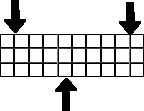# Physics - Numerical Methods

We could divide an object into a finite number of parts, in 3 dimensions, possibly a 4th dimension for time, and against this calculate some physical properties? forces, etc.In this way, the calculations can be simplified, even if there are more of them. The shape can be made up of cubes, each cube is the same size and mass.

#### Modeling Motion

If we consider a non-deformable case, then the velocities and rotations will be the same for each cube. But how do we calculate this velocity? I guess we need to calculate the forces on each cube to do this:

#### Modeling Forces

Each cube in the shape will experience different forces and torques. There will be forces due to fields (gravity, magnetic, etc.) and there will be forces and torques transmitted between the cubes through their faces. This is where the number crunching is needed, but at least dividing the shape into cubes simplifies the analysis. We need to work out the forces on each cube, the cubes on the outside are simpler as there cant be any force on an outside face (excluding air/fluid pressure, or fields) so a technique might be to compute the forces starting from the outside and working in.

#### Collision detection

This method might simplify collision detection? by holding the position of each cube, then if 2 or more cubes occupy the same position, then there is a collision.

#### Collision response

A collision will introduce a force between the colliding objects at the point of impact for an infinitesimally short time (impulse force) so this can be modeled using the same mechanism as other forces.

#### TorquesIt seems quite difficult to model an object subject to bending forces. Is it realistic to expect to be able to model bending in this way, This could be a good test of this model.

 metadata block see also: Correspondence about this page Book Shop - Further reading. Where I can, I have put links to Amazon for books that are relevant to the subject, click on the appropriate country flag to get more details of the book or to buy it from them.Flexible Multibody Dynamics: A Finite Element Approach There is also an e-book (Adobe Reader) version availableCommercial Software Shop Where I can, I have put links to Amazon for commercial software, not directly related to the software project, but related to the subject being discussed, click on the appropriate country flag to get more details of the software or to buy it from them.

This site may have errors. Don't use for critical systems.